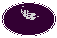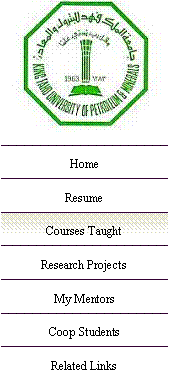Dr. Mazen M. Elrougi
 Assistant Professor of Systems Engineering – KFUPM
 Courses Taught
 Currently:   ISE - 325 : Engineering Statistics (Hypothesis testing, simple and multiple linear regression, statistical experiments and analysis)   ISE - 307 : Engineering Economy (Present and future value, rate of return on investments, IRR, CB analysis)   Previous Courses:   CISE - 301: Numerical Methods (Numerical integration and differentiation, solution to differential equations, interpolation and regression)   ISE - 201: Introduction to Industrial and Systems Engineering   Labs: ISE - 405: Simulation Modeling & Analysis ISE - 303: Linear Programming